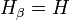# Descendant subgroup

This article defines a subgroup property: a property that can be evaluated to true/false given a group and a subgroup thereof, invariant under subgroup equivalence. View a complete list of subgroup properties[SHOW MORE]
If the ambient group is a finite group, this property is equivalent to the property: subnormal subgroup
View other properties finitarily equivalent to subnormal subgroup | View other variations of subnormal subgroup |
This is a variation of subnormality|Find other variations of subnormality |

## Definition

A subgroup$H$ of a group$G$ is termed descendant if we have subgroups$H_\alpha$ of$G$ for every ordinal$\alpha$ such that:

•$H_0 = G$
•$H_{\alpha + 1} \ \underline{\triangleleft} \ H_\alpha$ (i.e.,$H_{\alpha + 1}$ is a normal subgroup of$H_\alpha$) for every ordinal$\alpha$.
• If$\alpha$ is a limit ordinal, then$H_\alpha = \bigcap_{\gamma < \alpha} H_\gamma$.

and such that there is some ordinal$\beta$ such that$H_\beta = H$.

### In terms of the descendant closure operator

The subgroup property of being an descendant subgroup is obtained by applying the descendant closure operator to the subgroup property of being normal.

## Facts

### Descendant-contranormal factorization

This result states that given any subgroup$H$ of$G$, there is a unique subgroup$K$ containing$H$ such that$H$ is contranormal in$K$ and$K$ is descendant in$G$.

## Metaproperties

Metaproperty name Satisfied? Proof Statement with symbols
transitive subgroup property Yes descendance is transitive If$H \le K \le G$ are groups such that$H$ is a descendant subgroup of$K$ and$K$ is a descendant subgroup of$G$, then$H$ is a descendant subgroup of$G$.
trim subgroup property Yes Every group is descendant in itself, and the trivial subgroup is descendant in any group.
intermediate subgroup condition Yes descendance satisfies intermediate subgroup condition If$H \le K \le G$ are groups such that$H$ is descendant in$G$, then$H$ is descendant in$K$.
strongly intersection-closed subgroup property Yes descendance is strongly intersection-closed If$H_i, i \in I$, are all descendant subgroups of$G$, so is the intersection$\bigcap_{i \in I} H_i$.
image condition No descendance does not satisfy image condition It is possible to have groups$G$ and$K$, a descendant subgroup$H$ of$G$ and a surjective homomorphism$\varphi:G \to K$ such that$\varphi(H)$ is not a descendant subgroup of$K$.